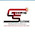• In DC circuit, power supply to the load is simply the product of voltage across the load and current flowing through it i.e., P= VI. Because in DC circuits, there is no conept of phase angle between current and voltage. In other words, there is no power factor in DC circuits.
• But the situation is Sinusoidal or AC Circuits is more complex because of phase difference between Current and Voltage.In AC circuits, When circuit is pure resistive, then the same formula used for power as used in DC as P = V I.

Types of power in AC circuits:
1. Real power
2. Reactive power
3. Apparent power
1.Real power (P):
• It is defined as the amount of power delivered from source to load.
• This component is responsible for generating heat (converts the Electrical Energy into Heat energy). True power gets consumed in the circuit as heat.

• Heat is dissipated in the resistive load.

2.Reactive power (Q):

• It is defined as the amount of power flowing back and forth between the source and load.
• As we know that, the charging elements like inductor and capacitors. The inductor(inductive load is highly used in practice) is responsible for creating a magnetic field and it doesn't generate any heat.
• Let's see the inductor( current lags behind the voltage by 90 degree) wave form.

• As you see the power wave form, during positive half cycle the inductor is charged and during negative half cycle the inductor discharges the stored energy.

3.Apparent power (S):

• The total power delivered to the load is called apparent power or we can say the combination of real power and reactive power.
• Total power in an AC circuit, both dissipated and absorbed/returned is referred to as apparent power.
• We can define these powers in simple triangle i.e in trigonometric form. This triangle is called as power triangle.

By Pythagoras theorem,

(Apparent power)^2 = (Real power)^2 + (Reactive power)^2.

NOTE: If you people want me to write any other topic, just put it in comment. It will be welcomed and updated within 48 hours.

#### 1 comment:

1.Very informative and useful historical post. I see you have a lot of other cool stuff available at your website, simply bookmarking it to check in spare time. Thanks and keep the good work up.

Pump Station Monitoring